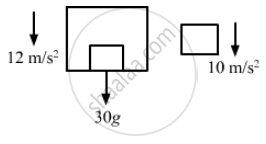# P a Block is Kept on the Floor of an Elevator at Rest. the Elevator Starts Descending with an Acceleration of 12 M/S2. Find the Displacement of the - Physics

Sum

A block is kept on the floor of an elevator at rest. The elevator starts descending with an acceleration of 12 m/s2. Find the displacement of the block during the first 0.2 s after the start. Take g = 10 m/s2.

#### Solution

The free-body diagram of the system is shown below:The two bodies are separated because the elevator is moving downward with an acceleration of 12 m/s2 (>g) and the body moves with acceleration, g = 10 m/s2 [Freely falling body]

Now, for the block:
g = 10 m/s2, u = 0, t = 0.2 s
So, the distance travelled by the block is given by
$s = ut + \frac{1}{2}a t^2$
$= 0 + \frac{1}{2}10 \times \left( 0 . 2 \right)^2 = 5 \times 0 . 04$
= 0 . 2 m = 20 cm
The displacement of the body is 20 cm during the first 0.2 s.

Concept: Newton’s Second Law of Motion
Is there an error in this question or solution?

#### APPEARS IN

HC Verma Class 11, Class 12 Concepts of Physics Vol. 1
Chapter 5 Newton's Laws of Motion
Q 42 | Page 83